Mathematics
Easy

Question

# Plot the data in a line plot.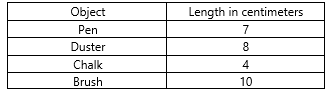##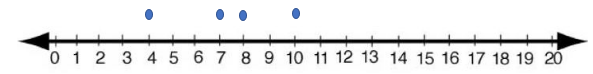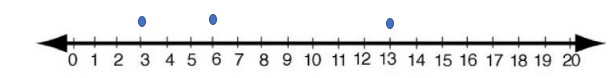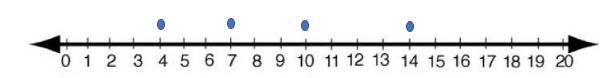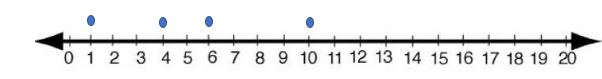Hint:

## The correct answer is:### A line plot is a graph that displays data using a number line. To create a line plot, ​first create a number line that includes all the values in the data set. Next, place an X (or dot) above each data value on the number line.*Given points are 7cm. 8cm, 4cm, 10cmStep 2: Next, place an X (or dot) above each data value on the number line.* From the line plot, we can see that the required line plot is given in option A.#### With Turito Foundation.#### Get an Expert Advice From Turito.